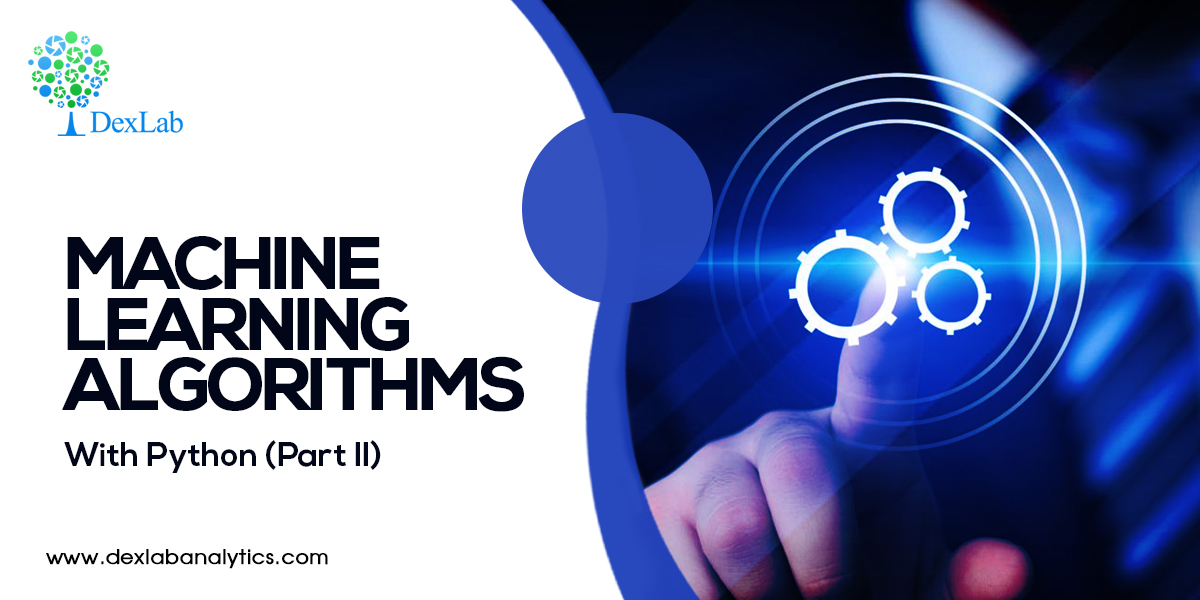# Machine Learning Algorithms – With Python (Part II)In the first part of this blog, we covered Parametric and Non-Parametric Machine Learning algorithms and Supervised and Unsupervised Machine Learning Algorithms. If you haven’t gone through it yet, check it out here: dexlabanalytics.com/blog/machine-learning-algorithms-with-python-part-i

In this blog we are going learn about Semi Supervised Machine Learning algorithms.

#### What are Semi Supervised ML algorithms?

Those algorithms in which only half of the historical data’s target data has been specified are called semi-supervised algorithms. The way to go about solving this is by making a model on the basis of the portion of historical data that has the target specified and then apply this model to the rest of the data to predict the outcomes. Now, combine the two sets of data, get the target variable and make a model on the basis of this target variable.

#### New Nomenclature

In the equation Y= B0 + B1X, Y is called the Target Variable while in statistics it is called the Dependent Variable. And X is called Features or Attributes whereas in statistics it is called Independent Variable. B0 and B1 are called Weights while in statistics they are called Coefficients (Intercept and Slope, respectively).

In the equation Ÿ – Y = error, the error in statistics is called Residual but in Machine Learning it is called Cost Function. And the elements of the historical data set that in statistics are known as Records or Observations, in machine learning are known as Instances.

#### What is Bias Variance Trade-Off?

In parametric algorithms like linear regressions, several assumptions are made before building a model. These assumptions can be things like having only those inputs that have a relationship with the target variable or the fact that the error should be random.  The benefit of this process is the fact that Ÿ or the predicted results are consistent and there is not much variance in them.Now, if we are to take a Decision Tree or any other non-parametric Machine Learning algorithm, a small change in the data set forces a large variance in the Target variable. But, unlike in parametric ML algorithms, there are no basic assumptions in non-parametric assumptions. So, in such a case, the error or mean square error, is a combination of the square of bias and variance.

MSE = Bias2 + Variance

Increasing any one (the square of the bias) will lead to a decrease in the other (variance) and vice versa.

In this case, we need to balance or trade off the two – the square of the bias and the variance.

While the bias cannot be changed much, we can control the variance by increasing or decreasing the parameters of the experiment.

#### What is Overfitting and Underfitting?

Overfitting is the condition when the accuracy figure of the ‘trained’ data set is larger in number than the accuracy figure of the ‘tested’ unseen data set. This is an undesirable condition. Underfitting is the opposite wherein the accuracy figure of the trained data is lower than that of the tested unseen data. This is also undesirable. What we seek to aim at is an equal accuracy in both the tested and trained models.

To limit Overfitting we must –

• Use a resampling technique to estimate model accuracy by repeating experiments with the data and then drawing an average of the accuracy figures.
• Hold back a validation data set to test your model on and increase the number of models to experiment on the trained data set.

We would like to conclude out second part of this tutorial here. For more on this, visit the third blog on Machine Learning Algorithms with Python.

(Translated from 28:00 – 1:19:00)

.

Comments are closed here.### Call us to know more### Gurgaon+91 931 572 5902